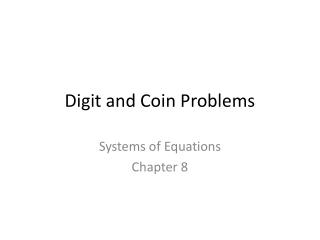Download PresentationDigit and Coin Problems

Loading in 2 Seconds...

# Digit and Coin Problems - PowerPoint PPT Presentation

Digit and Coin Problems. Systems of Equations Chapter 8. Any two digit number can be expressed as 10x + y. x represents the tens place and y represents the ones place . . 45. x=4 and y=5. 10(4) +(5) =. 45. 71. x=7 and y=1. 10(7) +(1) = . 71. 29. x=2 and y=9.I am the owner, or an agent authorized to act on behalf of the owner, of the copyrighted work described.
Download Presentation## Digit and Coin Problems

An Image/Link below is provided (as is) to download presentation

Download Policy: Content on the Website is provided to you AS IS for your information and personal use and may not be sold / licensed / shared on other websites without getting consent from its author.While downloading, if for some reason you are not able to download a presentation, the publisher may have deleted the file from their server.

- - - - - - - - - - - - - - - - - - - - - - - - - - E N D - - - - - - - - - - - - - - - - - - - - - - - - - -
Presentation Transcript
1. Digit and Coin Problems Systems of Equations Chapter 8

2. Any two digit number can be expressed as 10x + y x represents the tens place and y represents the ones place. 45 x=4 and y=5 10(4) +(5) = 45 71 x=7 and y=1 10(7) +(1) = 71 29 x=2 and y=9 10(2) +(9) = 29

3. The sum of the digits of a two digit number is 14. If the digits are reversed, the number is 36 greater than the original number. Find the original number. Let x = tens place y = ones place System of Equations x + y = 14 Equation1 Original Number 9x - y = -36 Equation 2 10x + y Reverse Number Reversed Number = Original Number + 36 10y + x 10y + x = 36 + 10x + y

4. Coins

5. Kami has some nickels and some dimes. The value of the coins is \$1.65. There are 12 more nickels than dimes. How many of each kind of coin does Kami have? nickels dimes Let n = # of Let d = # of System of Equations 5n + 10d = 165 Value n = d + 12 Quantity

6. There were 411 people at a play. Admission was \$5 for adults and \$3.75 for children. The receipts were \$1978.75. How many adults and how many children attended? Let a = # of Let c = # of adults children System of Equations a + c = 411 Quantity 5a + 3.75c = 1978.75 Value

7. Age Problems

8. Shirley is 21 years older than Laura. In six years, Shirley will be twice as old as Laura. How old are they now? Let x = Shirley’s age Let y= Laura’s age x + 6 = Shirley’s age in six years y + 6 = Laura’s age in six years Shirley in 6 years = 2 (Laura in 6 years) x + 6 = 2 (y + 6) System of Equations x = y + 21 Now x = 2y + 6 In 6 years